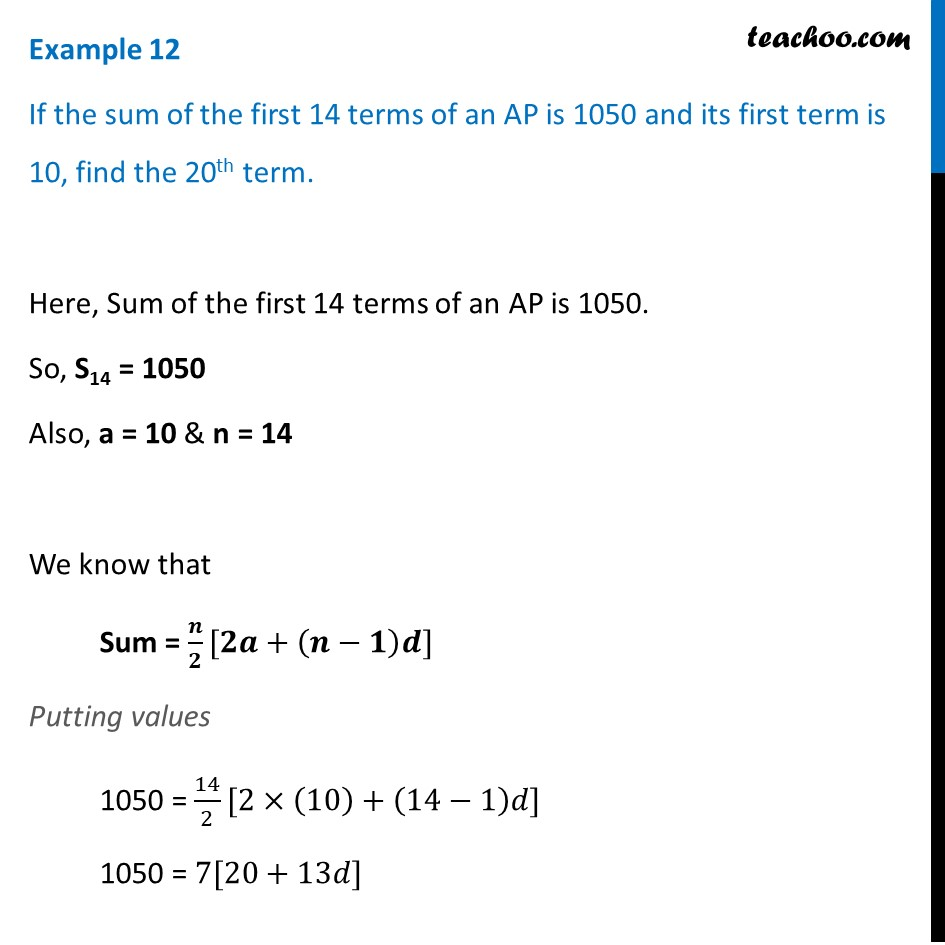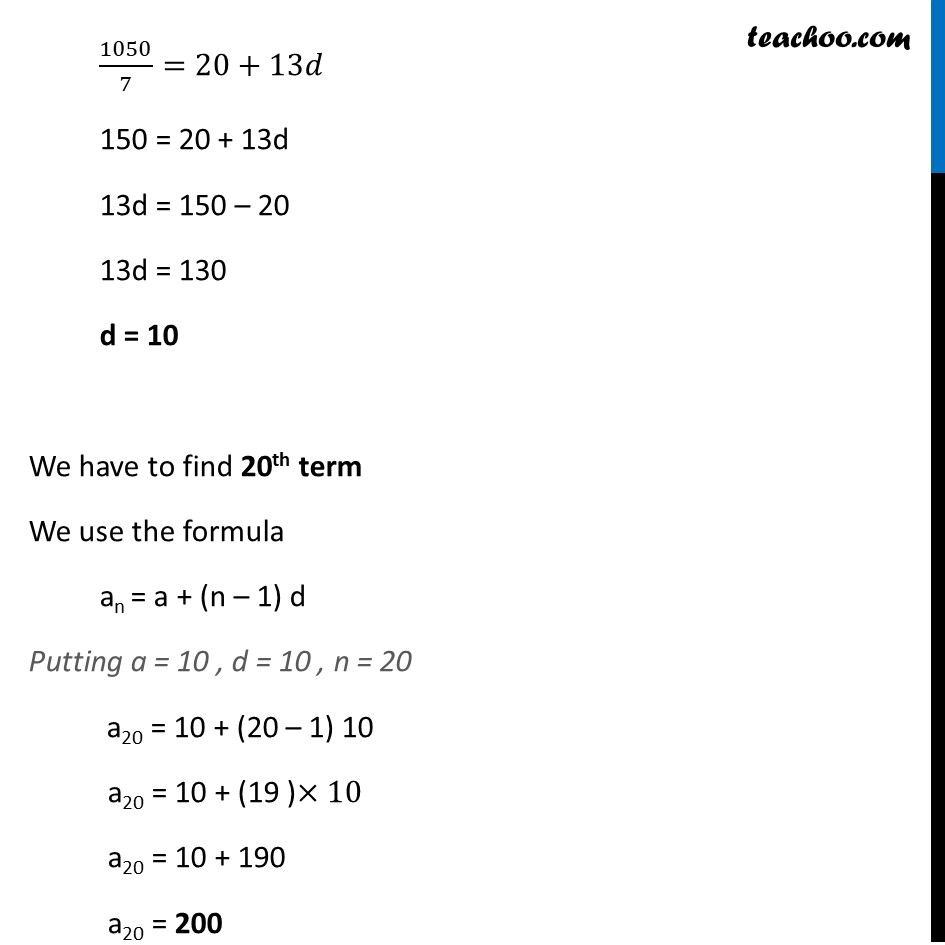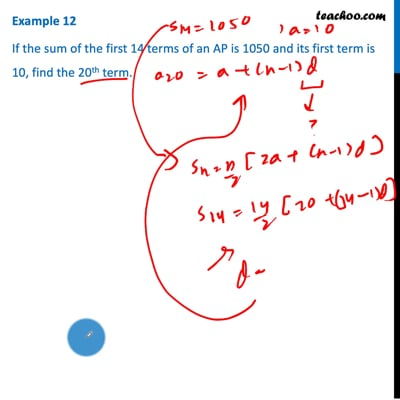Finding number of terms given s

Chapter 5 Class 10 Arithmetic Progressions
Concept wiseThis video is only available for Teachoo black users

Introducing your new favourite teacher - Teachoo Black, at only ₹83 per month

### Transcript

Example 12 If the sum of the first 14 terms of an AP is 1050 and its first term is 10, find the 20th term. Here, Sum of the first 14 terms of an AP is 1050. So, S14 = 1050 Also, a = 10 & n = 14 We know that Sum = 𝒏/𝟐[𝟐𝒂+(𝒏−𝟏)𝒅] Putting values 1050 = 14/2[2×(10)+(14−1)𝑑] 1050 = 7[20+13𝑑] 1050/7=20+13𝑑 150 = 20 + 13d 13d = 150 – 20 13d = 130 d = 10 We have to find 20th term We use the formula an = a + (n – 1) d Putting a = 10 , d = 10 , n = 20 a20 = 10 + (20 – 1) 10 a20 = 10 + (19 )×10 a20 = 10 + 190 a20 = 200